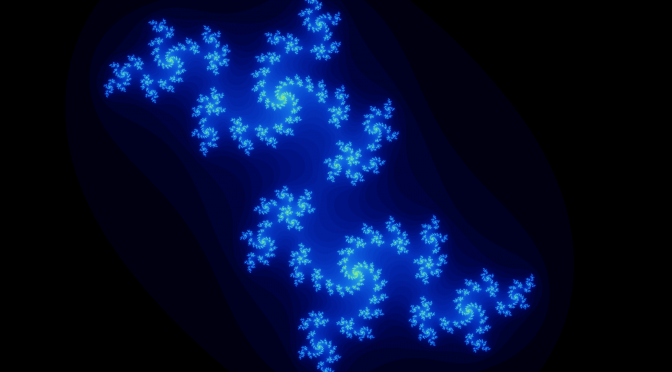Quite like I described the Mandelbrot set, the Julia set is also a Mathematical fractal that is computed with almost the exact iteration. The iteration isa quadratic polynomial in this case. Here,andare both complex numbers, but play a rather different role than in the Mandelbrot equation.

Going back to our original understanding of this iteration,was a constant mapped to a point in the set, anda value used for computation with an initial value of. Now, by repeating the iteration it was decided that ifthe point was not part of the Mandelbrot set. As the points inside the set will naturally not converge (for they are part of the set), the iteration is restricted to a finite number of recursions.

What separates the Julia set(s) is that the meanings ofandhave been swapped. Nowhas an initial value mapped to a point in the set andis now an arbitrary constant. Thus, there exist an infinite amount of Julia sets.

I will present the Julia set only in its form of a quadratic polynomial, as we will discover semblances to the Mandelbrot set when discussing the many variations.

### Some Julia sets

Let us start with the most simple form of the set when we set, thus reducing the iteration toWhat follows is a simple circle, also called the unit circle. It has a radius of one and sits at the origin.

A fine explanation for this figure is that any numberwill always to converge to zero (and thus be part of the set) ifThe entire set of this form are the points with this property.

When we increase the real value ofwe see a fractal of spirals emerge which appear to be mirrored both horizontally and vertically.

As we increase that value the spirals begin to distance themselves from each other and shrink. Notable is that the vertical distance grows faster than the horizontal one.

For larger imaginary values ofwe find another interesting spiral pattern. Hereby the spirals repeat inside each other infinitely. Atthe spirals begin to fade out and the figure looks more like branches connecting with each other. Similar to the shape before, the figure dissolves as the value becomes larger. If we now make the value negative we see the same pattern only mirrored horizontally.

When we assign negative real values towe find some astonishing similarities to the Mandelbrot set. Atthe figures becomes very much resembling of the Mandelbrot shape. The figure is again mirrored on both axes. Increasing that value in the negative direction condenses the fractal into lines, or branches. This looks similar to the tail of the Mandelbrot set.
But what about the Mandelbrot set? All of the figures presented can be indeed found inside the Mandelbrot set, as the Mandelbrot set is actually the sum of all possible Julia sets. Astounding, isn’t it? This is because the Mandelbrot set at each point has a different value of. And that is why all Julia sets (in the form of a quadratic polynomial) combined form the Mandelbrot set.
I have vaguely hinted before, but the Julia set may have different iteration types. For example there isor even! The Internet will gift you with astonishing videos and images about these at no cost at all.